1. If tan A and tan B are the roots of the quadratic equation $x^{2}-px+q=0$    , then value of $\sin^{2}\left(A+B\right)$    is
a) $\frac{p^{2}}{p^{2}+q^{2}}$
b) $\frac{p^{2}}{\left(q+p\right)^{2}}$
c) 1 - $\frac{p}{\left(1-q\right)^{2}}$
d) $\frac{p^{2}}{\left(1-q\right)^{2}+p^{2}}$

Explanation: We have tan A + tan B = p, and tan A tan B = q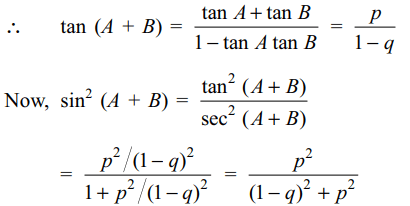2. The real value of a for which the sum of the squares of the roots of the equation $x^{2}-\left(a-2\right)x-a-1=0$      assume the least value is
a) 0
b) 1
b) 2
c) 3

Explanation: Discriminant of the equation is3. If p and q are distinct primes and $x^{2}-px+q=0$     has distinct positive integral roots, then p + q equals
a) 5
b) 7
c) 19
d) 40

Explanation: Let $\alpha$ and $\beta$ be two roots of x2– px + q = 0 and4. The real values of a for which the quadratic equation $3x^{2}+2\left(a^{2}+1\right)x+\left(a^{2}-3a+2\right)=0$        possesses roots of opposite signs lie in
a) $\left(-\infty,1\right)$
b) $\left(-\infty,0\right)$
c) $\left(1,2\right)$
d) $\left(\frac{3}{2},2\right)$

Explanation: The quadratic equation $3x^{2}+2\left(a^{2}+1\right)x+\left(a^{2}-3a+2=0\right)$ will have two roots of opposite signs if it has real roots and the product of the roots is negative, that is, if5. If $a,b,c \epsilon R$   and a + b + c = 0, then the quadratic equation $3ax^{2}+2bx+c=0$     has
a) at least one root in [0, 1]
b) at least one root in [1, 2]
c) at least one root in [3/2, 2]
d) none of these

Explanation: Let f(x) = ax3 + bx2 + cx. Note that f is continuous and derivable on R. Also f(0) = 0 and f(1) = a + b + c = 0. By the Rolle’s theorem, there exists at least one $\alpha$ $\epsilon$ (0, 1) such that6. Let $f\left(x\right)=ax^{2}+bx+c,a,b,c\epsilon R$       and $a\neq 0$   . Suppose f (x) > 0 for all $x \epsilon R$ . Let$g\left(x\right)=f\left(x\right)+f'\left(x\right)+f''\left(x\right)$      . Then
a) $g\left(x\right)>0 \forall$   $x \epsilon R$
b) $g\left(x\right)< 0 \forall$   $x \epsilon R$
c) $g\left(x\right)=0 \forall$    $x \epsilon R$
d) g(x) = 0 has real roots

Explanation: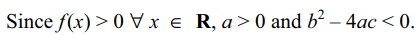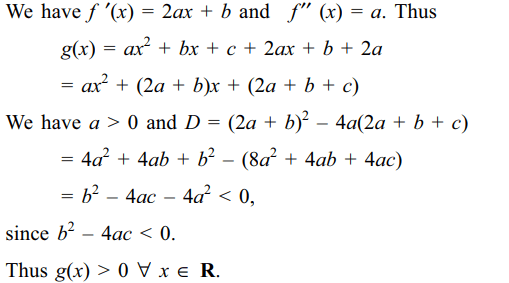7. If b < 0, then the roots $x_{1}$ and $x_{2}$ of the equation $2x^{2}+6x+b=0$     , satisfy the condition $\left(x_{1}/x_{2}\right)+\left(x_{2}/x_{1}\right)< k$      where k is equal to.
a) -3
b) -5
c) -6
d) -2

Explanation: The discriminant of the quadratic equation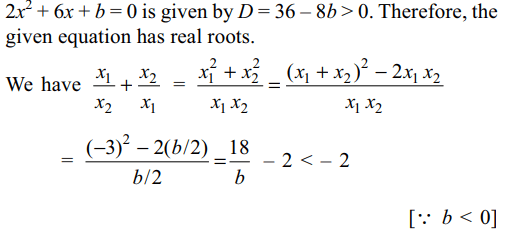8. If $ax^{2}+bx+c,a,b,c \epsilon R$       has no real zeros, and if c < 0, then,
a) a < 0
b) a+b+c>0
c) a > 0
d) none of these

Explanation: Let f (x) = ax2 + bx + c. Since f (x) has no real zeros, either f (x) > 0 or f (x) < 0 for all x $\epsilon$ R. Since f (0) = c < 0, we get f (x) < 0 $\forall$ x $\epsilon$ R. Therefore, a < 0 as the parabola y = f (x) must open downwards.

9. If x is real, then the maximum value of $y=2 \left(a-x \right)\left(x+\sqrt{x^{2}+b^{2}}\right)$
is
a) $a^{2}+b^{2}$
b) $a^{2}$
c) $\sqrt{a^{2}+b^{2}}$
d) $a\sqrt{a^{2}+b^{2}}$

Explanation: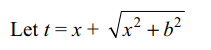10. If both the roots of the equation $x^{2}-6ax+2-2a+9a^{2}=0$      exceed 3, then
a) a < 1
b) a > 11/9
c) a > 3/2
d) a < 5/2